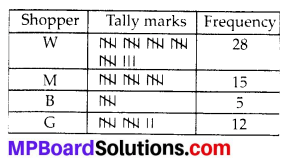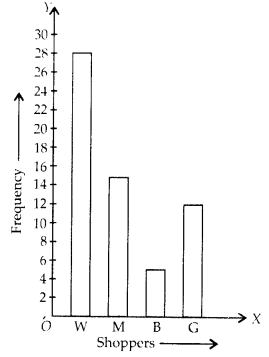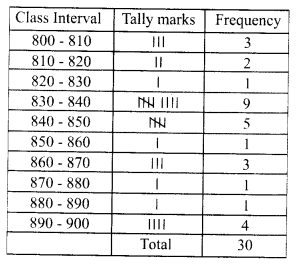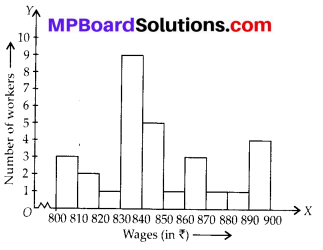# MP Board Class 8th Maths Solutions Chapter 5 Data Handling Ex 5.1

## MP Board Class 8th Maths Solutions Chapter 5 Data Handling Ex 5.1

Question 1.
For which of these would you use a histogram to show the data?
(a) The number of letters for different area in a postman’s bag.
(b) The height of competitors in an athletics meet.
(c) The number of cassettes produced by 5 companies.
(d) The number of passengers boarding trains from 7:00 a.m. to 7:00 p.m. at a station. Give reasons for each.
Solution:
(a) We use pictograph for the given statement. Since, letters can be represented through appropriate picture or symbols, we will not use histogram.
(b) We use histogram for the given statement. Since, the height of competitors in an athletics meet can be divided into class intervals.
(c) We will not use histogram. We use pictograph for the given statement since the cassettes can be represented through appropriate picture or symbols.
(d) We use histogram for the given statement since the time of boarding trains from 7:00 a.m. to 7:00 p.m. can be divided into class intervals.Question 2.
The shoppers who come to a departmental store are marked as : man (M), woman (W), boy (B) or girl (G). The following list gives the shoppers who came during the first hour in the morning:

WWWGBWWMGGMMWWWWGBMWB
GGMWWMMWWWMWBWGMWWWW
GWMMWWMWGWMGWMMBGGW
Make a frequency distribution table using tally marks. Draw a bar graph to illustrate it.
Solution:
Firstly by using a given information, we make a frequency distribution table using tally marks.
Shopper Tally marks FrequencyQuestion 3.
The weekly wages (in ₹) of 30 workers in a factory are:
830, 835, 890, 810, 835, 836, 869, 845, 898, 890,820,860,832,833,855,845,804,808,812, 840,885,835,835,836,878,840,868,890,806, 840.
Using tally marks make a frequency table with intervals as 800 – 810, 810 – 820 and so on.
Solution:
By using a given information, we have to make a frequency table :Question 4.
Draw a histogram for the frequency table made for the data in Question 3, and answer the following questions.
(i) Which group has the maximum number of workers?
(ii) How many workers earn ? 850 and more?
(iii) How many workers earn less than ? 850?
Solution:
We have to draw a histogram :(i) 830 – 840 is a group which has the maximum number of workers.
(ii) 10 workers earn ₹ 850 and more.
(iii) 20 workers earn less than ₹ 850.Question 5.
The number of hours for which students of a particular class watched television during holidays is shown through the given graph. Answer the following:
(i) For how many hours did the maximum number of students watch TV?
(ii) How many students watched TV for less than 4 hours?
(iii) How many students spent more than 5 hours in watching TV?
Solution:(i) The above given graph shows that in between 4-5 hours, maximum number of students used to watch TV.
(ii) Number of students watched TV for less than 4 hours = 4 + 8 + 22 = 34
(iii) Number of students spent more than 5 hours in watching TV = 8 + 6 = 14